# 23 Deep Learning Papers To Get You Started — Part 1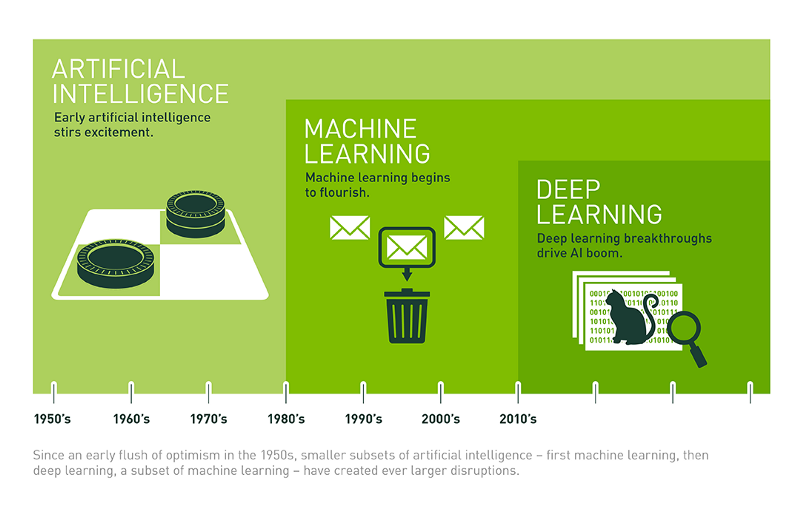Source : NVIDIA

Deep Learning has probably been the single-most discussed topic in the academia and industry in recent times. Today, it is no longer exclusive to an elite group of scientists. Its widespread applications warrants that people from all disciplines have an understanding of the underlying concepts, so as to be able to better apply these techniques in their field of work. As a result of which, MOOCs, certifications and bootcamps have flourished. People have generally preferred the hands-on learning experiences. However, there is a considerable population who still give in to the charm of learning the subject the traditional way — through research papers.

Reading research papers can be pretty time-consuming, especially since there are hordes of publications available nowadays, as Andrew Ng said at an AI conference, recently, along with encouraging people to use the existing research output to build truly transformative solutions across industries.

In this series of blog posts, I’ll try to condense the learnings from some really important papers into 15–20 min reads, without missing out on any key formulas or explanations. The blog posts are written, keeping in mind the people, who want to learn basic concepts and applications of deep learning, but can’t spend too much time scouring through the vast literature available. Each part of the blog will broadly cater to a theme and will introduce related key papers, along with suggesting some great papers for additional reading.

In the first part, we’ll explore papers related to CNNs — an important network architecture in deep learning. Let’s get started!

### 1. A Few Useful Things to Know about Machine Learning — Pedro Domingos

I thought we should start with a refresher on ML. This paper provides 11 handy tips/lessons, equally applicable to machine learning and deep learning.

• Learning = Representation + Evaluation + Optimization :
Representation is choosing the right set of classifiers/hypotheses, e.g. : k-NN, Naive Bayes, Propositional rules, Bayesian networks etc.
Evaluation deals with scoring function development to distinguish good classifiers from bad ones, e.g. Accuracy, Precision, K-L divergence etc.
This is followed by choosing the most efficient optimization technique for the given representation, e.g. Greedy search, Gradient descent etc.
• Avoid overfitting/Generalization is important :
It’s important to set aside a test/holdout dataset and perform cross-validation while training your model. Generalization error can be decomposed into bias and variance. Bias is the tendency of the learner to constantly learn the same wrong thing, whereas, Variance is the tendency to learn the noise (wrong labelling, sampling error) in the data. Regularization, getting more data, performing statistical significance tests, such as chi-square are the solutions.
• Data alone is not enough :
Every learner must embody some prior knowledge or assumptions (smoothness, similar examples having similar classes, limited complexity etc.), beyond the data it is given in order to use induction and generalize beyond it.
• More data > cleverer algorithm
This is essentially because all algorithms have the same target and follow similar basic approaches. For example, propositional rules are encoded within ANNs. Many algorithms use feature similarity-based approach.
• Intuition fails in higher dimensions/ Curse of dimensionality :
Increase in dimension increases the search space exponentially. A large number of training examples constitute only a fraction of the input space now, making generalization difficult. Similarity-based reasoning, which many ML algorithms depend on, break down in higher dimensions, since now, a lot of different examples have close similarity scores or are “nearby”. Human intuition fails with visualization beyond 3-D.
• Feature engineering is the key :
Feature engineering is the key difference between average and good classifiers. It takes up the maximum time in an ML project, but is the most important step. More and more of the feature engineering process is being automated nowadays.
• Learn many models/ Ensemble learning :
Combining variations from multiple models give a big boost to the learner accuracy. Bagging involves generating random variations of the training set by re-sampling, learning a classifier on each, and combining the results by voting. In boosting, a new function is added to the learner at every step, which focus on the training examples, the previous version of learner had got wrong. Stacking is a multi-level learning methodology, where the outputs of the individual models become input to the next layer of models.
• Theoretical guarantees are not what they seem :
The main role of theoretical guarantees in ML is not as a criterion for practical decisions, but more as a source of understanding the driving force behind the algorithm design. This is because theoretically, lots of assumptions are made and the bounds are loose.
• Simplicity does not imply accuracy :
This flies straight in the face of Occam’s Razor. In model ensembles (boosting), the generalization accuracy improves on adding new functions/classifiers. An SVM can effectively have infinite parameters (making the learner complex), without overfitting.
• Representable does not imply learnable :
Many representations claim to be able to cater to all kinds of functions. However, they might still not be learnable. For example, standard decision tree learners cannot learn trees with more leaves than training examples. If the hypothesis space has many local optima of the evaluation function, the learner may not find the true function inspite of being representable.
• Correlation != Causation :
Learners/classifiers learn correlations between the individual features and the target variable. However, it is wrong to treat them as representing causal relationships. ML models are based on observational data, where the predictive variables are not under the control of the learner. Some algorithms can potentially extract causal connections, but their applicability is restricted.

### 2. Introduction to CNNs — Jianxin Wu

This is more of an article which helps the reader understand all the nitty-gritties of a CNN from a mathematical viewpoint. It starts with an explanation of tensors, vectorization, chain rule and then talks about the architecture of a CNN, all its layers (convolution, activation, pooling, loss) along with the training methodology of stochastic gradient descent (SGD) by applying back-propagation, ending with VGG-16, a highly useful CNN architecture.

Tensors can be thought of as, nothing but higher-order matrices. A tensor is to a matrix what a cube is to a square. A color image is represented as an order 3 tensor (H X W X 3) in height-width-channels format. Tensors can be easily vectorized and are highly used in different layers of a CNN.

Chain rule is utilized in the learning process of a CNN. The general notation is : dz/dx = (dz/dy).(dy/dx)
Here, z is a function of y, which in turn is a function of x. We apply the chain rule to get the derivative of z with respect to x using y in between. We’ll see how this rule is applied in back-propagation in a bit.

A CNN takes a tensor as an input, which is then processed sequentially, through a number of layers using the weight/parameter tensors.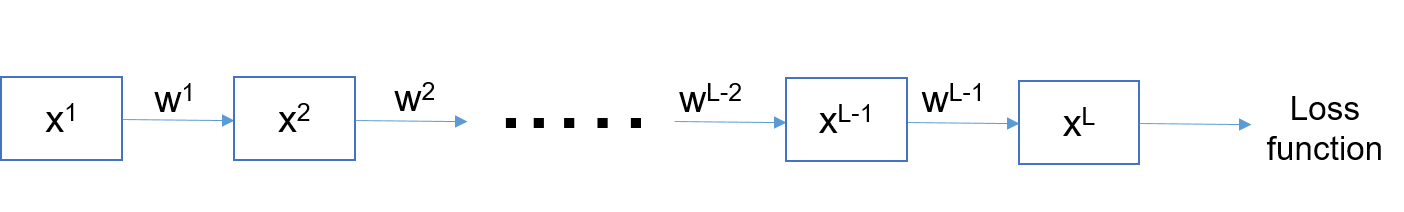Basic structure of a CNN — x¹, x² etc. are the tensor inputs and different layer responses; w¹, w² etc. are the learnable parameters/weights

Consider an object recognition problem with C target classes. The target output, y, will be a 1-D array of size C, having all the elements as zero except the correct class having a value of one. The loss layer is used to measure the error between the predicted class probabilities and y.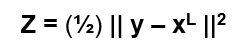z represents the error term/squared loss function

The layer just before the loss layer is a softmax function, which converts the network predictions to a probability mass function of the C target classes. L2 squared loss for regression and cross-entropy loss for classification are used. Prediction using a CNN only requires a forward pass, where the input, x¹ is processed through the various layers (using the learned weights w¹, w²…) to arrive at an estimated posterior probability distribution of x¹ for the C categories. The class/category with the maximum probability is predicted.

Stochastic Gradient Descent : Training the parameters of a CNN is done through gradient descent, an iterative optimization process which identifies the direction of steepest descent (the gradient) in an n-dimensional hyperplane (n is the number of parameters). It can be imagined as a ball being allowed to roll on a hill. All these are done to minimize the cost function/loss.

The loss z, acts as a supervisory signal and guides the updation procedure of the model parameters. The weights are reduced by the magnitude of gradient at a point, scaled by the learning rate. The general equation used is as below :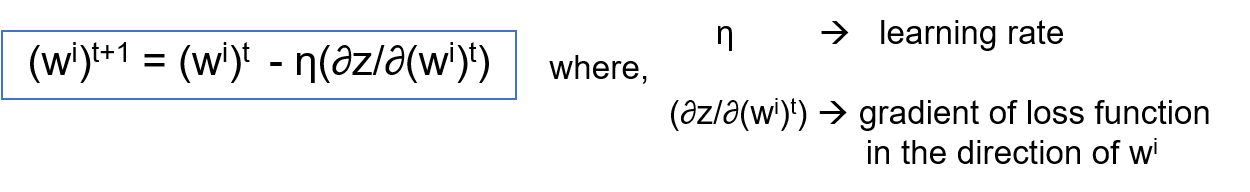Equation for weight updation at epoch t

The learning rate controls the movement of the weight vectors. Too high learning rate, and you might overshoot the minima/trough and the optimization process might diverge, i.e. cost function will start increasing. Keep too less a learning rate, and the optimization might take a long time to converge or get entrenched in a local minima. At a time, one training example is used to update the parameters. An epoch is an entire cycle of updation using all the training examples (either individually, or in batches).

We can also update the parameters using gradient estimated from a subset of training examples. This is called stochastic gradient descent (SGD). An extreme form of this is the batch gradient descent, where all the training examples are used for a single parameter update. In contrast, the usual practice is : mini-batch SGD where batches of training examples (in the power of 2) are used for single parameter updation. Mini-batch SGD is faster, converges quicker than batch gradient descent (requires less no. of epochs) and avoids overfitting.

Error back-propagation : The backpropagation algorithm is an approach to find how much a particular weight at any layer is responsible for the total loss and hence, the amount by which it should be modified. The error is propagated from the loss layer to the previous layers through two sets of partial derivatives/gradients — (i) gradient of the loss function with respect to weights at each layer, (ii) gradient of the loss function with respect to layer outputs at each layer. We use chain rule to compute these gradients.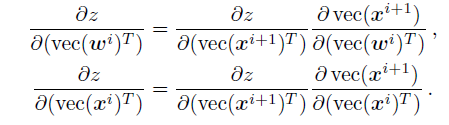The error is propagated layer by layer using the second gradient equation. The first is used to update the weights in layer i

Next, we look at the different layers in a convolutional neural network.

Convolution layer : To understand convolution layer, we need to answer the following questions — “What is convolution?”, “ Why to convolve?” and “How to back-propagate errors in convolution layers?”

What is convolution?
Convolution operation involves overlapping of a kernel of fixed size over the input tensor and then sliding across pixel-by-pixel to cover the entire image/tensor. For the overlapped area, we compute the product between the elements of the kernel and the image at the same location and then sum it up.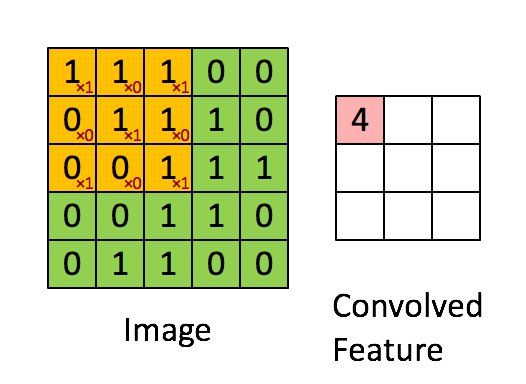Convolution operation in action. Source : giphy.com

The spatial extent of the output is smaller than that of the input if the convolution kernel is larger than 1X1. To ensure that the input and output tensors have the same size, we can apply zero padding (padding the input image all around by zeros). For example, in the above gif, a 5X5 tensor is reduced to a 3X3 tensor by a 3X3 kernel. Instead, if we add one row of zeros to the top and bottom, along with one column of zeros to the sides of the image, the output will be a 5X5 feature map, thus maintaining the input image size.

Another important concept in convolution is stride. Stride is basically the number of steps by which the convolution kernel slides each time. Generally, a stride of 1 is used as in the above animation. However, if stride, s > 1, convolution is performed once every s pixels, both horizontally and vertically.

In general, output size for a conv. layer is given by : O = (I-(F-S)+2P)/S
where, O is the output size, I is the input size, F is the filter/kernel size, P is the number of rows/columns padded and S is the stride.

Why to convolve?

• Convolution uses various filters, trained using backpropagation, to recognize simple patterns (edges, corners etc.) in images. In deeper layers, multiple feature detectors combine to detect complex patterns or objects.
• It helps the CNN in extracting features with local information. Topology of the input is not entirely ignored. This helps specially in audio and images.
• Parameter sharing/ weight replication by the convolution kernel helps in achieving shift invariance and also reduces the number of parameters.

Back-propagating errors in convolution layer :
Backpropagation in convolution layers follows a similar approach as in fully connected/dense layers. They use the chain rule to calculate partial derivative of the loss with respect to the output layer multiplied by partial derivative of output layer with respect to the convolution filter/kernel. Gradient of loss with respect to the conv filter is also calculated, to be used in weight updation.

Consider x to be the input layer, y the output layer, F the conv filter and z to be the loss function. Then with * as the convolution operator and x tilde as the row/column flipped version of the input,

The video below explains the same in detail.

ReLU layer : ReLU activation function is denoted as : relu(x) = max(0,x).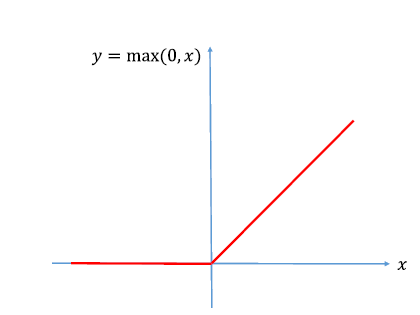The ReLU function

Importance of ReLU activation :

• It adds non-linearity to the CNN model. The relationship between semantic features of an image and its pixel values are obviously highly non-linear. ReLU helps in modelling that non-linearity to some extent.
• By truncating the negative feature map values to 0 and keeping only the positive values as it is, the ReLU function activates only for certain object patterns at some particular regions. Combining many such object parts’ detection helps in classifying the correct target class
• ReLU’s gradient being 1 for the activated features, helps in SGD learning. Compare that with a sigmoid or tanh activation functions, where the problem of vanishing gradients makes SGD difficult. Moreover, gradient calculation in ReLU is faster than sigmoid or tanh, speeding up the entire learning process considerably.

Pooling layer : Pooling is a local operator, which is applied individually on each image channel. The spatial extent (H X W) and the stride of the pool are a part of the design of CNN structure. The most commonly used pooling setup is a 2X2 (HXW) region with a stride of 2. The pooling layer encodes the neighbourhood information of a region into a single pixel.

• This helps in achieving a bit of invariance (positional + rotational)
• Pooling reduces the size of the receptive field significantly, thus reducing the training time, avoiding overfitting etc.

There are two kinds of pooling — max pooling and average pooling. Max pooling captures the highest activation value in a sub-region. Average pooling takes the mean of all activation values in the concerned sub-region. This results in feature detection with a rough idea of its location. Though we lose some information about the feature’s exact position, we gain a lot through the highly reduced size of the feature maps.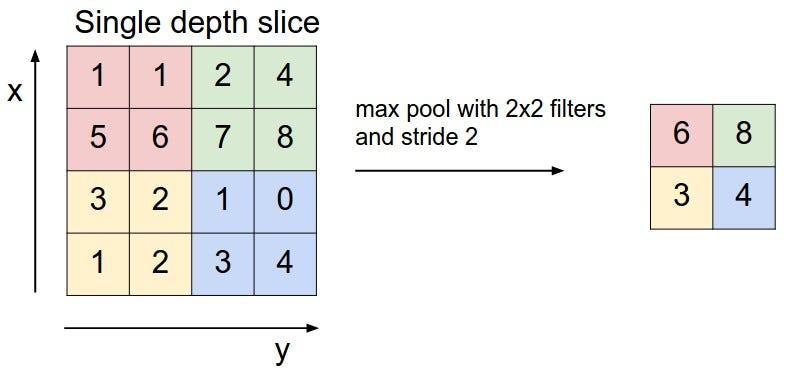Source : CS231n lectures. Max-pooling divides the image into small sub-regions and then extracts only the useful information from them to transmit further down the network

#### VGG-16 Net :

The paper ends with a brief description of Oxford VGG group’s “VGG Verydeep-16” model architecture. It is designed using the following layers :

• Convolutional layer : Convolutional layers with a filter size of 3X3, padding of 1 (same padding) and a stride of 1 is used. Due to a padding of 1, the convolutional layer keeps the size of the input tensor the same.
• ReLU : ReLU layers are used in VGG to model non-linearity in images.
• Pooling layer : Max pooling of kernel size 2X2 and a stride of 2 is used. This reduces the receptive field size by a factor of 2.
• Fully connected layer : The output of the last convolutional block (Conv-ReLU-Pool) is flattened and connected to a layer of neurons similar to hidden layers in an ANN. Represented as n1 X n2, (input X output size)
• Dropout layer : Dropout is a technique where a certain fraction of weights in the network are set to zero during training to improve the generalization ability of the model. In VGG-16, a fraction of 0.5 is used.
• Softmax layer : Softmax layer is used to convert the output to estimated posterior probability distribution of the various target classes.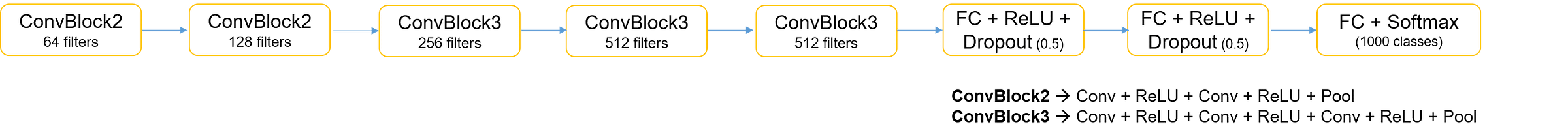VGG-16 architecture. All conv blocks have 3X3 kernel with same padding and a stride of 1

Stacking two 3X3 convolution layers is equivalent to one 5X5 convolution layer. Stacking three 3X3 such layers gives receptive field equivalent to one 7X7 layer. The advantage of stacking multiple 3X3 layers over larger size kernels is that it results in lesser number of learnable parameters and thus reduces the chances of overfitting. Also, computation time is reduced.

VGG-16 takes an image with size 224 X 224 X 3 as an input and is trained on the ImageNet dataset, an object recognition problem with 1000 classes. Pre-trained VGG-16 models can be used for transfer learning, but care has to be taken that the input images are similar to the ones in ImageNet dataset.

### 3. Visualizing and Understanding Convolutional Networks — Matt Zeiler, Rob Fergus

This is the famous paper (ZF Net),which unravelled the black box of CNNs by visualizing the outputs of intermediate layers of a convolutional network. This was a huge step forward in building interpretable deep learning models. In and around 2012, we had powerful GPUs, large labelled training sets, new model architectures and regularization techniques like Dropout. Convolutional nets became the go-to architecture for object detection and image classification tasks. However, how they worked remained a mystery.

Through this paper, Zeiler and Fergus aimed to introduce a visualization technique that would reveal the exact input stimuli that excite feature maps in the intermediate layers by projecting the feature activations back to input pixel space. A visualization module would also shed more insights regarding which parts of the image are important for classification, how do the features evolve as we train the models etc. This is done using a Deconvolutional network. Prior to this, standard convnet models (AlexNet type) were trained on the ImageNet dataset. The model layers were convolved with a set of learnable filters, passed through a ReLU unit and max pooling over local neighbourhoods was performed to build the feature maps. Optionally, batch normalization was also done to normalize the responses across feature maps.

A deconvnet, consisting of : (i) unpooling, (ii) rectification and (iii) filtering operations was attached to each layer. In unpooling, a set of switch variables are used to record the maxima locations within each pooling region in the convnet architecture. These switches are then used by the deconvnet model to obtain an approximate inverse by placing the reconstructions of the activations in the feature map to the appropriate locations in the layer below.
Rectification process simply involves passing the reconstructed signal through a ReLU non-linearity. In the convnet model, the feature maps are passed through ReLU activations, thus ensuring positive values as : ReLU = max(0,x). If x is positive, the ReLU function transforms to a linear function (y = x), whose inverse is the same linear function. Thus, passing the reconstructed signal through ReLU in the deconvnet model makes sense.
In the filtering stage, the deconvnet model uses the transposed versions of the learned filters used by the convnet earlier and applies it to the rectified maps. An explanation for why the transposed version is used can be found below.

The switches settings in the deconvnet are specific to individual images. Thus, the reconstruction obtained from a single activation resembles a small, but discriminative part of the input image.

Feature visualization of the trained model is performed by projecting the top 9 activations each of various feature maps down to pixel space to reveal the structures/patterns that excite a particular feature map. Corresponding image patches from ImageNet validation set are also shown to understand more about the object/part of scene being explained by the model.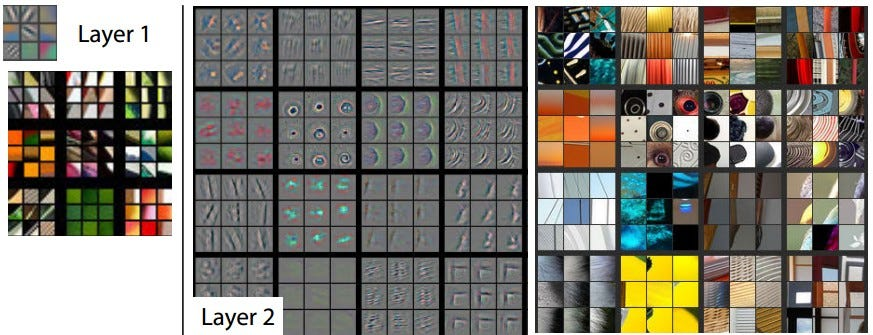Feature visualizations : Layer 1 captures edges and color gradients in different orientations, while Layer 2 is a notch higher, capturing corners and patterns formed from Layer 1 features; Source: Image taken from Zeiler and Fergus’s ECCV 2014 paper

Some insights obtained from using the visualization technique :

• The layers learn hierarchical features. Lower level layers (layer 1 and 2) learn edges, color gradients, corners and edge-color conjunctions. Higher layers build off the features in the previous layers with layer 3 capturing texture and text patterns, layer 4 capturing animal body parts (faces, legs) and layer 5 capturing entire object (dogs, keyboards etc.) in different poses. Higher layers capture more complex invariances.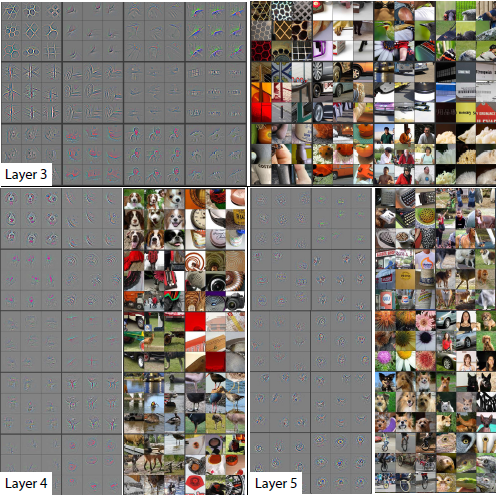Higher layers combine features from previous layers and capture different forms of invariance (pose, scene location etc.); Source : Zeiler, Fergus, ECCV 2014 paper
• The lower layers of the model converge only after a few epochs. But, the higher layers require atleast 40–50 epochs to build useful feature representations.
• The network output is stable to translations and scaling, but in general invariant to rotation. The first layer is highly sensitive to small transformations (translations, rotations, scaling), while the robustness to these effects build up as we move to higher layers.
• The first couple of layers miss out on mid-frequency information in images and show aliasing effects due to large stride in the first convolutional layer of AlexNet. These are corrected by reducing the first layer filter size from 11X11 to 7X7 and making the stride 2, instead of 4.

Experiments for occlusion sensitivity and checking correspondence between specific object parts in different images were also performed. Different portions of the input image are covered by a grey square and passed through the network, with the classifier output being monitored. It was seen that the probability of the correct class dropped significantly when the object of interest in the image was occluded. Specifically, there is also a strong drop in the activation value of the feature maps which were earlier triggered by this object or image part. For correspondence analysis, 5 dog images with frontal pose were taken and the same part of the face was masked out in each. The difference between feature vectors from the original and occluded image is calculated and the consistency between these difference vectors (for all image pairs) is calculated using Hamming Distance. A lower value of this metric indicates tighter correspondence between same object parts in different images. The model, thus, showed that it was able to capture the correct, discriminative regions of the image for classification.

More experiments were done to find the ideal depth of the network. It was seen that removing a couple of layers, here and there, didn’t reduce the performance much, but removing a combination of a few convolutional and fully-connected layers worsened the model accuracy dramatically. Deeper feature hierachies also captured more discriminative features.

The ZF Net network, thus built, was trained on ImageNet 2012 datasets and reported a lower error rate than the best performing model of that time. The convolutional block part of the network turn into effective feature extractors and this helps in generalizing to other image datasets, like Caltech-101 and Caltech-256. Pascal VOC dataset has multiple objects in their images and are different in nature from ImageNet. Being pre-trained on ImageNet, ZF Net was not able to beat the state-of-the-art for Pascal VOC 2012 dataset.

Zeiler and Fergus’s model approach was important in encouraging the growth of more interpretable deep learning models. In the next part of this series, we’ll explore papers explaining key concepts towards optimization, learning and regularization in DL models. All the papers mentioned in this article and more are also available at this Github repo.